# Math Operations

Math Operations blocks perform arithmetical operations on numbers.

Error values, and text that cannot be resolved to numbers, cause a NaN error result to be returned. The following are accepted and excluded arguments for Math Operations blocks:

• Numbers, logical values, and numbers represented as strings are accepted.
• Error values, and text that cannot be resolved to numbers, are ignored in the calculation.
Block Icon Description
Add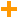Adds its arguments
Divide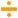Divides its arguments
Factorial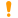Returns the single factorial of a number
Logarithm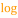Calculates the logarithm of a number using a specified base
Modulo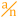Returns the quotient and remainder from division
Multiply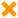Multiplies its arguments
Power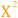Returns the result of raising a number to an exponent
Root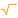Returns the nth root of a number
Scale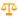Returns the result of re-scaling a number from one defined set of lower and upper limits to another.
Subtract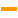Subtracts its arguments

2018/10/03 00:17